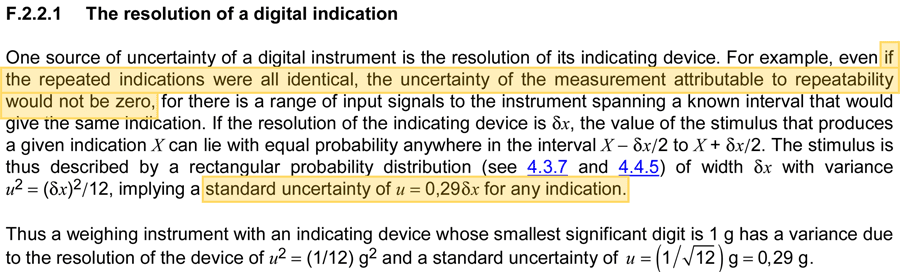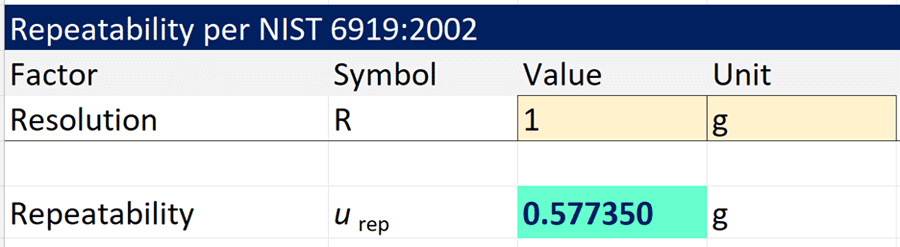# Repeatability is Zero, Here’s What to Do## When Repeatability is Zero

Once in a while, you may encounter a scenario where your measurement repeatability is zero. This is common for certain types of measurements, such as:

• measuring a gauge block with a caliper,
• placing a calibrated mass on a scale or balance, or
• calibrating a handheld DMM with a multifunction calibrator.

In fact, I see this quite often. I have clients collect repeatability and reproducibility data for their uncertainty budgets and the results will have no variability.

Typically, this is the result of one or more of the following factors:

1. a lack of UUT resolution,
2. a very stable artifact, reference standard, or reference material,
3. very stable environmental conditions,
4. a consistent measurement process, and(or)
5. well trained personnel.

If you have encountered this situation and wondered what to do, you are in luck. This guide is going to show you what to, plus give you supporting evidence from industry recommended guides (so you can defend yourself during an audit).

Here is what is covered in this guide:

1. Repeatability Cannot Be Zero
2. What the GUM Recommends
3. Recommendations from Other Guides
4. Calculate Repeatability from Instrument Resolution
5. Important Considerations

## Quick Summary

Don’t have time to read the full article? Here is what you need to know in a quick summary.

When repeatability is zero, calculate repeatability based on the instrument resolution using one of the following methods:

• Method A (JCGM 100): Divide instrument resolution by the square root of 12, or
• Method B (NISTIR 6919): Divide instrument resolution by the square root of 3.

## Repeatability Cannot Be Zero

Now, you may think this is not a problem because your measurement process has no variability. However, you will have a problem if you list repeatability is zero in your uncertainty budget. Most assessors will not accept zero for repeatability.

In fact, the NISTIR 6919 states;

• it is possible to make seven or more measurements with every measurement resulting in the same value,” and
• standard deviation of zero is not statistically possible, though the standard deviation may be less than one display increment (d).

From these statements, you can conclude that:

1. Repeatability cannot be zero, and
2. A standard deviation of zero is not statistically impossible.

Additionally, the JCGM 100:2008 states, “…even if the repeated indications were all identical, the uncertainty of the measurement attributable to repeatability would not be zero

So, while you may think zero repeatability is great, you may be setting yourself up for an audit deficiency.

If you have encountered this situation and wondered what to do, I have a solution for you.

## What the GUM Recommends

Occasionally, people ask me what to do when their repeatability is zero. Typically, I recommend what is recommended in the JCGM 100:2008, the GUM.

According to the GUM, when repeatability is zero, you should calculate repeatability based on the resolution of the instrument. To do this, divide the resolution (R or d) by the square root of 12 which is equivalent to:

1. dividing the resolution by two times the square root of 3, or
2. multiplying the resolution by 0.29.

In Appendix F, section F.2.2.1, the GUM states:

One source of uncertainty of a digital instrument is the resolution of its indicating device. For example, even if the repeated indications were all identical, the uncertainty of the measurement attributable to repeatability would not be zero, for there is a range of input signals to the instrument spanning a known interval that would give the same indication. If the resolution of the indicating device is δx, the value of the stimulus that produces a given indication X can lie with equal probability anywhere in the interval X − δx/2 to X + δx/2. The stimulus is thus described by a rectangular probability distribution (see 4.3.7 and 4.4.5) of width δx with variance u2 = (δx)2/12, implying a standard uncertainty of u = 0,29δx for any indication.”

In the image below, you will see the excerpt from the JCGM 100:2008, Section F.2.2.1.## Recommendations from Other Guides

Another common recommendation, similar to the GUM, is given in the NISTIR 6919 guide.

The NISTIR 6919 recommends dividing the resolution by the square root of three. If you want to use the divide by the square root of 12 method (from the GUM), you must first perform a discrimination test to confirm the value is likely to lie near the center of the resolution interval.

Since most people do not perform this discrimination test, it is more common to use the divide by the square root of three method. For simplicity, this is commonly referred to as 0.577R of 0.577d.

In the image below, you will see the excerpt from the NISTIR 6919.## Calculate Repeatability Based on Instrument Resolution

If you conduct an R&R (i.e. Repeatability and Reproducibility) study and the results of your repeatability is zero, then follow one of the methods below to calculate repeatability based on the resolution of the unit under test (UUT).

1. Method A: Divide by the Square Root of 12 (JCGM 100:2008), and
2. Method B: Divide by the Square Root of 3 (NISTIR 6919)

### Method A: Divide Resolution by the Square Root of 12

If you follow the GUM recommendation when repeatability is zero, then you need to calculate repeatability by dividing the instrument resolution by the square root of 12.

#### Formula

Below, you will see the formula to calculate repeatability uncertainty.Where,
urep – Uncertainty due to repeatability (k=1),
R – Instrument Resolution

You may notice two options. You can either:

1. Divide the Resolution by the square root of 12, or
2. Multiply the resolution by 0.29.

Both formulas will give you the same result. So, pick the formula that is best for you.

#### Instructions

To calculate repeatability based on the resolution of an instrument, follow the instructions below:

1. Find the instrument resolution (R),
2. Divide the resolution by the square root of 12, or multiply the resolution by 0.29.The result will be your repeatability uncertainty. You can enter it into your uncertainty budget and characterize it with a normal distribution at 68% confidence or k=1.

If you notice the lower-case “u” in the formula, that will let you know that the uncertainty is at a one standard deviation level or k=1.

### Method B: Divide Resolution by the Square Root of 3

If you follow the NISTIR 6919 recommendation when repeatability is zero, then you need to calculate repeatability by dividing the instrument resolution by the square root of three.

#### Formula

Below, you will see the formula to calculate repeatability uncertainty.Where,
urep – Uncertainty due to repeatability (k=1),
R – Instrument Resolution

You may notice two options. You can either:

1. Divide the Resolution by the square root of 3, or
2. Multiply the resolution by 0.577.

Both methods will give you the same result. So, pick the method that is best for you.

#### Instructions

To calculate repeatability based on the resolution of an instrument, follow the instructions below:

1. Find the instrument resolution (R),
2. Divide the resolution by the square root of 3, or multiply the resolution by 0.577.The result will be your repeatability uncertainty. You can enter it into your uncertainty budget and characterize it with a normal distribution at 68% confidence or k=1.

If you notice the lower-case “u” in the formula, that will let you know that the uncertainty is at a one standard deviation level or k=1.

## Important Considerations

When calculating repeatability uncertainty based on the instrument resolution, there are several considerations that you should take into account.

Most importantly, the three things that you should consider are:

• Acceptable use of these methods,
• Reasonable use of these methods, and
• Not a substitute for repeatability testing.

### Acceptable Use of these Methods

Acceptable use of the methods in this guide is debatable. While the JCGM 100:2008 (GUM) recommends dividing the resolution by the square root of 12, other documents and professionals recommend dividing the resolution by the square root of three.

Objective evidence in the GUM should satisfy acceptable use, but the opinions of others may not accept the application of the method recommended by the GUM.

Notably, the NISTIR 6919 recommends dividing the resolution by the square root of 3 unless you have performed a discrimination test (per NIST Handbook 44 or OIML R-76) that can justify the use of dividing it by the square root of 12. However, the GUM (i.e. JCGM 100:2008) is the most recommended and followed guide for estimating uncertainty in measurement.

Acceptable use of either method is subject to professional opinions which makes it a debatable topic. If questioned, make sure to refer to the appropriate documents that support your evaluation. Otherwise, it may not be accepted. When in doubt, dividing the resolution by the square root of three is the most widely accepted method and would carry less risk of a deficiency.

### Reasonable Use of these Methods

The methods in this guide should be used only in reasonable scenarios.

Generally, it is reasonable to use these methods to evaluate repeatability when:

1. The standard deviation of repeated measurements is zero, or
2. making repeated measurements is not practical, cost-effective, or feasible.

For example, per the NISTIR 6919, a large capacity scale may not require evaluating repeatability using repeated measurements. Therefore, the methods in this guide would be reasonable for use.

Other examples and scenarios are given in the NISTIR 6919 and the EURACHEM CG4 guides.

### Not a Substitute for Repeatability Testing

The methods in this guide are meant to evaluate a measurement process only when repeatability is zero. It is not considered a substitute for conducting repeatability and reproducibility studies unless it meets the reasonable use criteria in the previous section.

There are plenty of labs that only evaluate repeatability based on instrument resolution and have done so for years without being cited a deficiency. With many assessors requesting objective evidence of repeatability records, it may be a matter of time before these labs get a deficiency during an audit.

Therefore, make sure you are conducting R&R studies (if it is reasonable to do so) and recording the results. If your repeatability is zero, then use one of the methods in this guide.

## Conclusion

Uncertainty due to repeatability cannot be zero. It is not statistically possible.

When repeatability is zero, you must use an alternative method to estimate uncertainty due to repeatability.

In this guide, you should have learned how to evaluate repeatability uncertainty using instrument resolution in accordance with both the:

• JCGM 100:2008 – Guide to the Expression of Uncertainty in Measurement, and
• NISTIR 6919 – Guide for Determining and Reporting Uncertainties for Balances and Scales

Both methods are an ideal solution to evaluate repeatability and avoid getting a deficiency for reporting repeatability as zero in your uncertainty budget.

Next time you perform a repeatability test and all your results are the same (i.e. no change or variation), use one of the methods in this guide and make a note listing reference to the method used.

I hope this guide helps you. Leave a comment below and let me know which method you use and why.

Posted in: